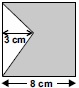# User Forum

Subject :IMO    Class : Class 7

Find the area of the shaded part in the square.A48 cm2
B52 cm2
C58 cm2
D64 cm2

## Ans 1:

Class : Class 7
As the length of side of square is 8 cm ,so it's area will be 8×8cm which is equal to 64 sq. cm.Now ,to find the area of triangle,i.e. the unshaded area ,we have to apply the formula of (1\2×base×height).Now the base is equal to the length of the side of square I.e. 8cm and the height is 3cm.Now the area of triangle is (1\2×8×3)cm =12cm.Now we have to subtract the unshaded area from the area of whole square I.e. 64 sq.cm-12 sq.cm =52sq.cm.So the answer is option B.

## Ans 2:

Class : Class 7
As the length of side of square is 8 cm ,so it's area will be 8×8cm which is equal to 64 sq. cm.Now ,to find the area of triangle,i.e. the unshaded area ,we have to apply the formula of (1\2×base×height).Now the base is equal to the length of the side of square I.e. 8cm and the height is 3cm.Now the area of triangle is (1\2×8×3)cm =12cm.Now we have to subtract the unshaded area from the area of whole square I.e. 64 sq.cm-12 sq.cm =52sq.cm.So the answer is option B.